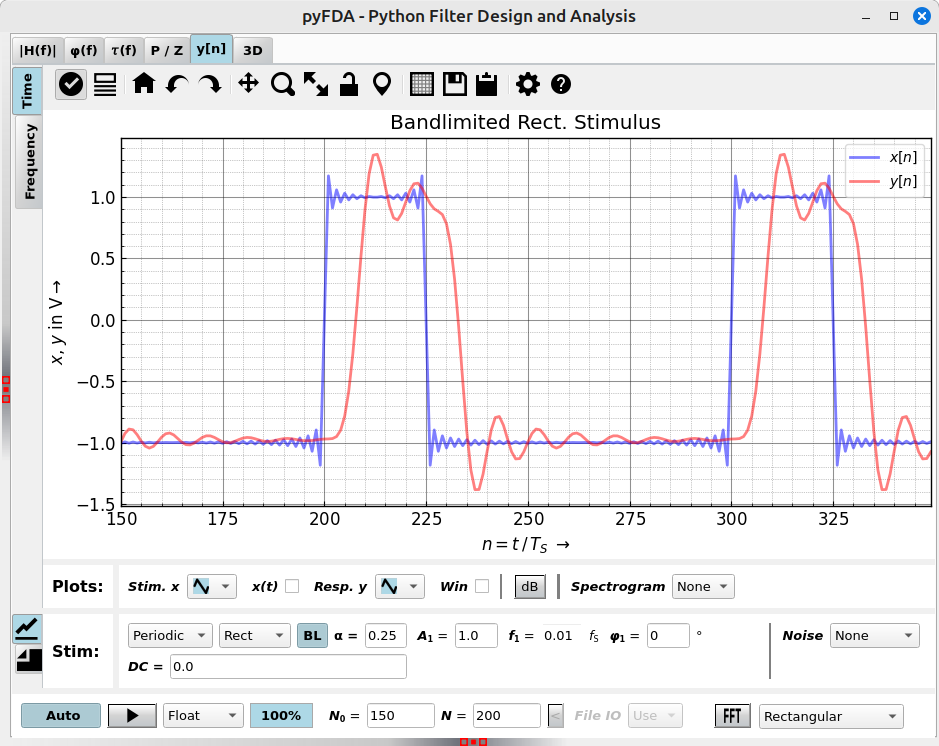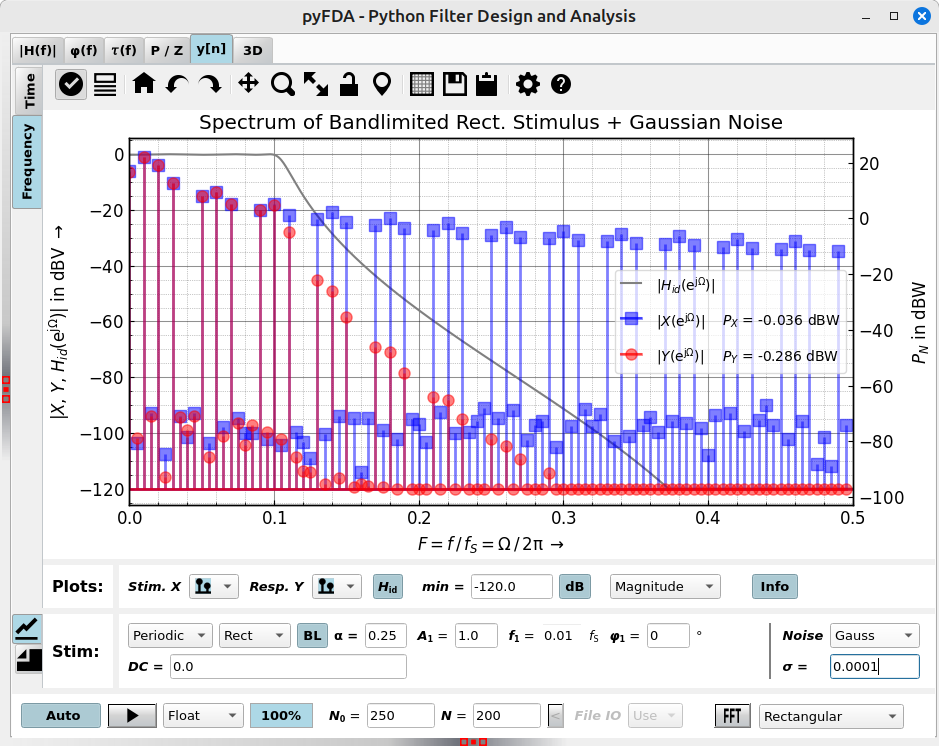# Plot h[n]¶

Fig. 22 shows a typical view of the h[n] tab for plotting the transient response and its Fourier transformation, here, an elliptical filter (IIR) is shown.Fig. 22 Screenshot of the h[n] tab (time domain)

There are a lot of options in this tab:

Time / Frequency

These vertical tabs select between the time (transient) and frequency (spectral) domain. Signals are calculated in the time domain and then transformed using Fourier transform. For an transform of periodic signals without leakage effect, (“smeared” spectral lines) take care that:

• The filter has settled sufficiently. Select a suitable value of N0.
• The number of data points N is chosen in such a way that an integer number of periods is transformed.
• The FFT window is set (in the Frequency tab) to rectangular. Other windows work as well but they distribute spectral lines over several bins. When it is not possible to capture an integer number of periods, use another window as the rectangular window has the worst leakage effect.
View
What will be plotted and how.
Stimulus

Select the stimulus, its frequency, DC-content, noise … When the BL checkbox is checked, the signal is bandlimited to the Nyquist frequency. Some signals have strong harmonic content which produces aliasing. This can be seen best in the frequency domain (e.g. for a sawtooth signal with f = 0.15). The stimulus options can be hidden with the checkbox Stim. Options.

DC and Different sorts of noise can be added.

Run
Usually, plots are updated as soon as an option has been changed. This can be disabled with the Auto checkbox for cases where the simulation takes a long time (e.g. for some fixpoint simulations)

The Fourier transform of the transient signal can be viewed in the vertical tab “Frequency” (Fig. 23). This is especially important for fixpoint simulations where the frequency response cannot be calculated analytically.Fig. 23 Screenshot of the h[n] tab (frequency domain)